# For the circuit given below, obtain the equations to analyze the circuit using chord current method...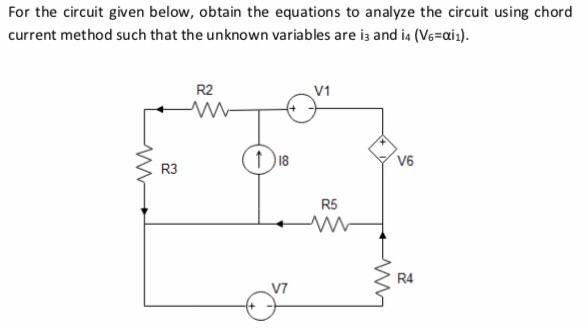For the circuit given below, obtain the equations to analyze the circuit using chord current method such that the unknown variables are i3 and ia (Ve-ai R2 V1 18 R3 R5 R4 V7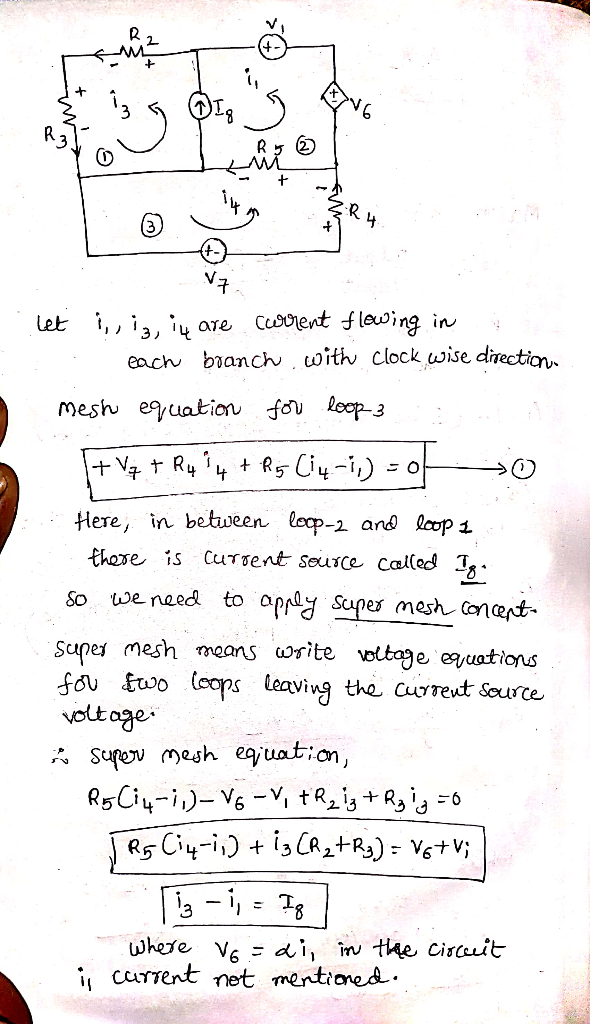#### Earn Coin

Coins can be redeemed for fabulous gifts.

Similar Homework Help Questions
• ### for the first equation, it will be v1. 5.(a) An electric circuit has five resistances and two applied voltages. Kirchoff's laws applied to 18] the circuit gives the following equations: -R4 + (R2...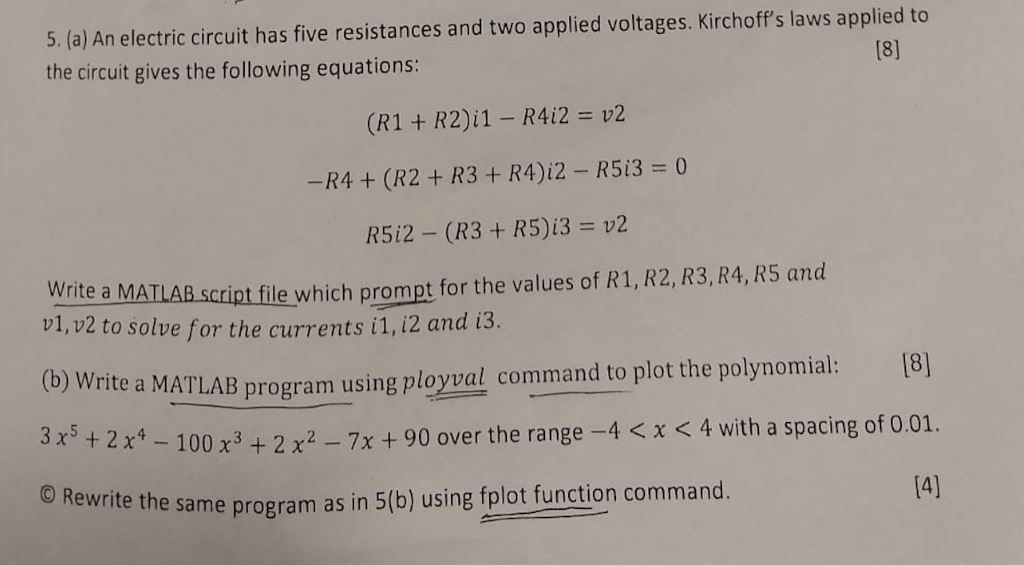for the first equation, it will be v1. 5.(a) An electric circuit has five resistances and two applied voltages. Kirchoff's laws applied to 18] the circuit gives the following equations: -R4 + (R2 + R3 + R4)i2-R513-0 R5i2-(R3 + R513 = v2 Write a MATLAB scipt file which prompt for the values of R1, R2, R3,R4, R5 and v1, v2 to solve for the currents i1, i2 and i3. ite a MATLAB program using ployval command to plot the polynomial:[8...

• ### Re-arrange the below circuit to calculate the current into R5 using current divider rule. No mark...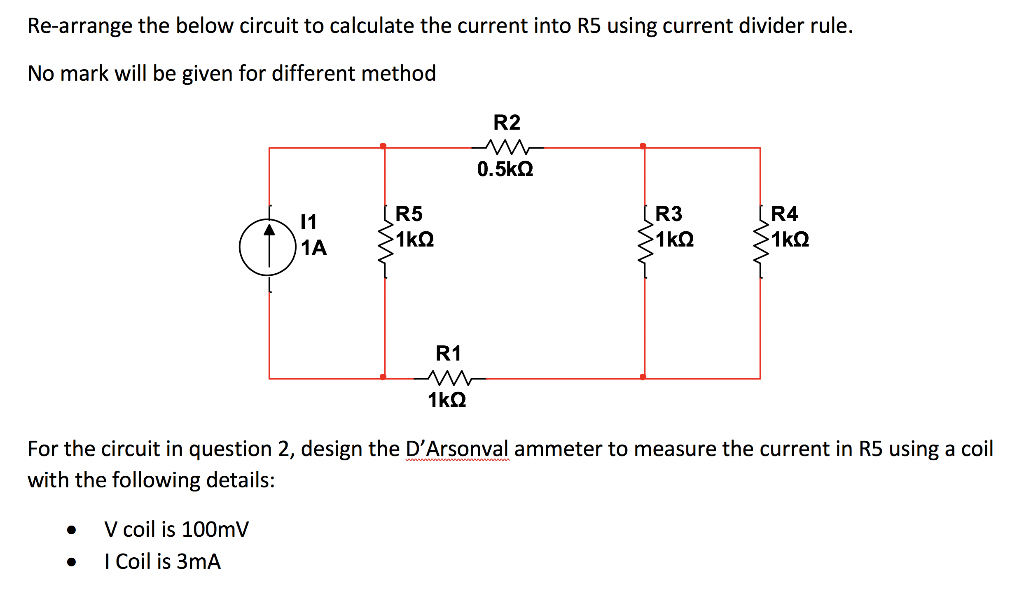Re-arrange the below circuit to calculate the current into R5 using current divider rule. No mark will be given for different method R2 0.5kQ R3 1kQ R4 1kQ R5 1kQ 1 1A R1 1kQ For the circuit in question 2, design the D'Arsonval ammeter to measure the current in R5 using a coil with the following details: V coil is 100mV I Coil is 3mA

• ### (TCO 4) For the circuit given in the figure below, obtain the following quantities. (a) Currents...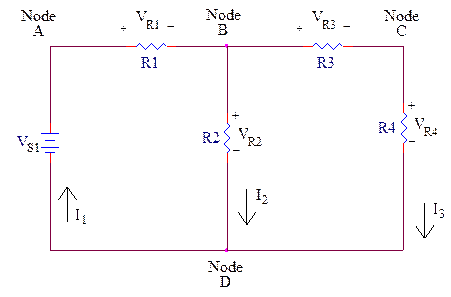(TCO 4) For the circuit given in the figure below, obtain the following quantities. (a) Currents I1 and I3 (b) Power dissipated by the resistor R2 Assume VS1 = 15 V, R1 = 4.0 k?, R2 = 9.0 k?, R3 = 6.0 k?, R4 = 12.0 k?

• ### For the circuit shown below, using Ohm's law enter in an equation for IR1, IR2, IR3...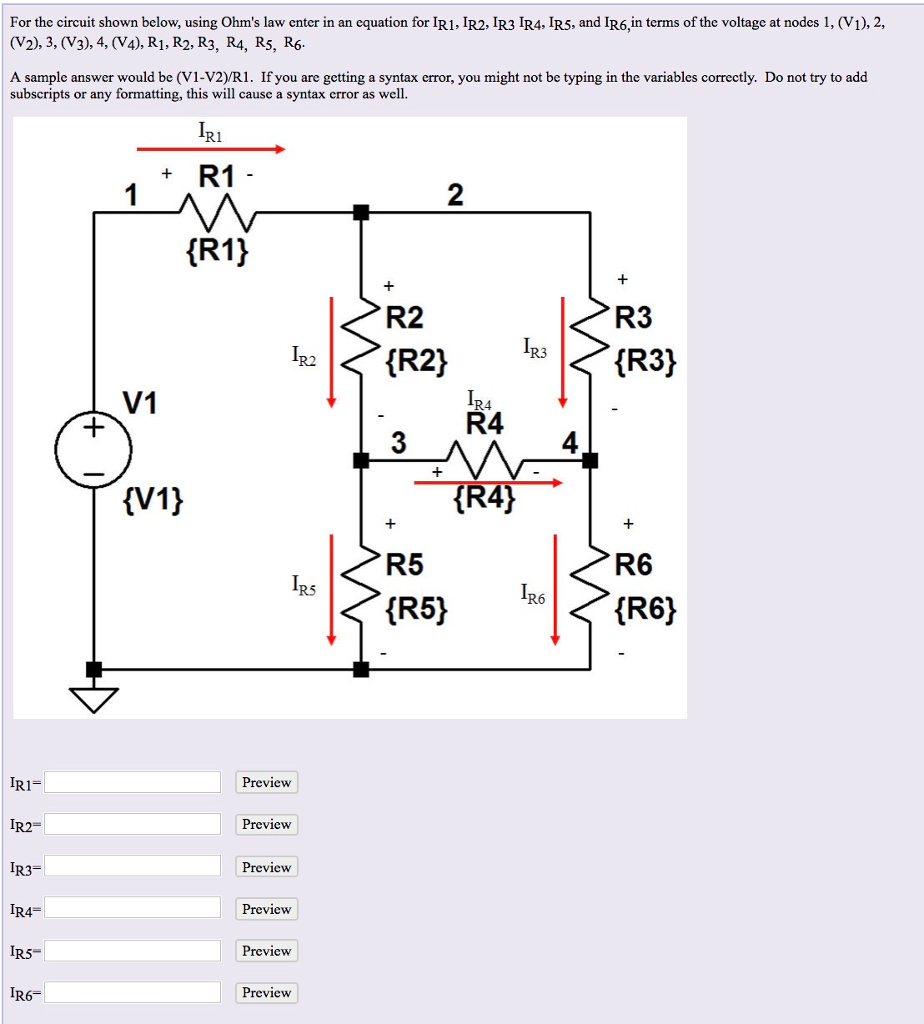For the circuit shown below, using Ohm's law enter in an equation for IR1, IR2, IR3 IR4, IR5, and IR6,in terms of the voltage at nodes 1, (V1),2, (V2), 3, (V3), 4, (V4), R1, R2, R3, R4, R5, Rf6 A sample answer would be (V1-V2)yR1. If you are getting a syntax error, you might not be typing in the variables correctly. Do not try to add subscripts or any formatting, this will cause a syntax error as well. R1 R1...

• ### Use the technique of simplifying this circuit using equivalent resistances into smaller, simpler circuits Given the...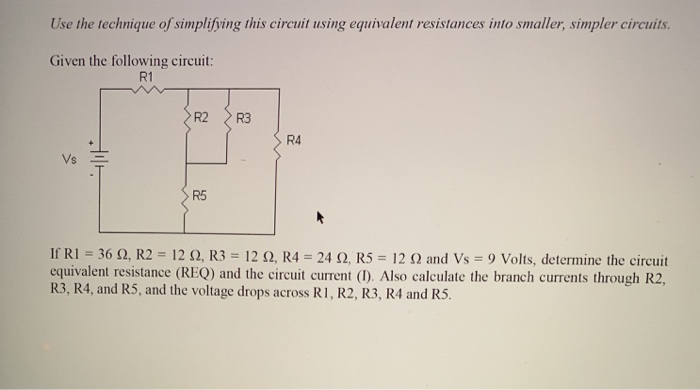Use the technique of simplifying this circuit using equivalent resistances into smaller, simpler circuits Given the following circuit: R1 R2 R3 R4 Vs E R5 If RI-36 Ω, R2 : 12 Ω, R3-12 Ω, R4 24 Ω, R5-12 Ω and Vs-9 Volts, determine the circuit equivalent resistance (REQ) and the circuit current (). Also calculate the branch currents through R2, R3, R4, and R5, and the voltage drops across R I, R2, R3, R4 and RS.

• ### Given the circuit on the diagram below with R1 = 6 kΩ, R2 = 13 kΩ,...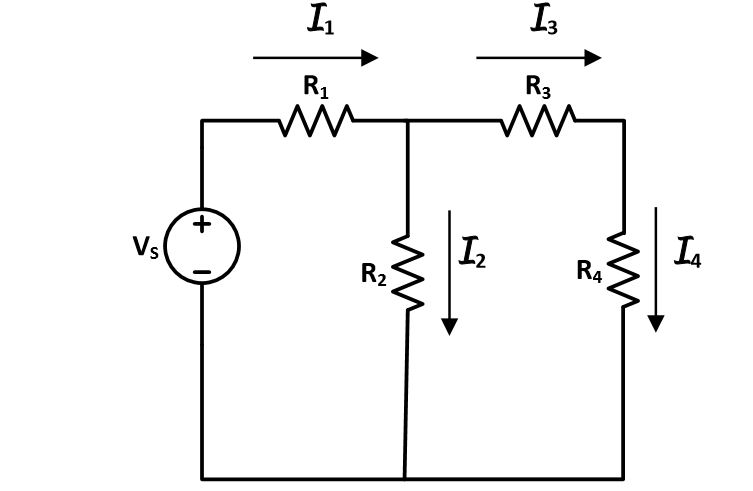Given the circuit on the diagram below with R1 = 6 kΩ, R2 = 13 kΩ, R3 = 11 kΩ and R4 = 9 kΩ. Using current and voltage division, find I1, I2, I3, and I4 , and also the voltages across the resistors (V1, V2, V3, and V4) when: Vs= 6 − 3cos100t V

• ### Show all work and explain answer 16) Given the circuit below, write ALL of Kirchhoff's Equations....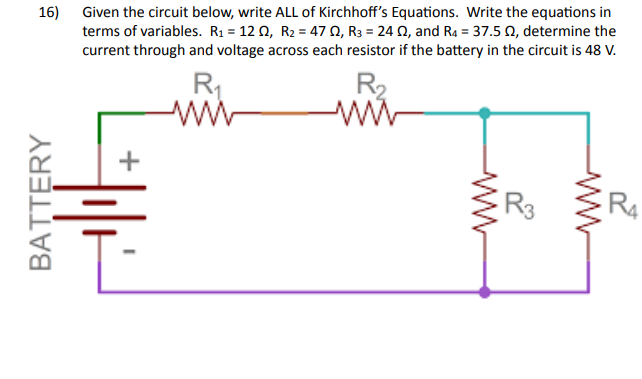Show all work and explain answer 16) Given the circuit below, write ALL of Kirchhoff's Equations. Write the equations in terms of variables. R1 = 12 N, R2 = 4712, R3 = 24 N, and Ra = 37.5 N, determine the current through and voltage across each resistor if the battery in the circuit is 48 V. R1 R W BATTERY Til R3 R4

• ### Perform nodal analysis on the circuit shown below in Figure 5 to find the unknown node voltage equations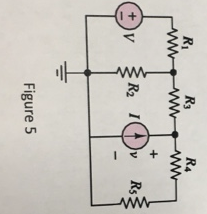5. Perform nodal analysis on the circuit shown below in Figure 5 to find the unknown node voltage equations. Let V = 3 V; I = 0.5 A; R1 = 20 Ω; R2 = 30Ω; R3 = 10Ω; R4 = 30Ω; R5 = 20Ω.

• ### Using the Loop Current Method, find all currents through the resistors in the circuit below CET...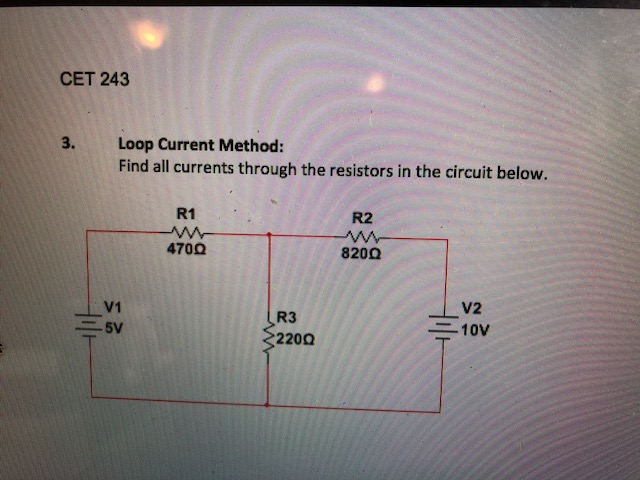Using the Loop Current Method, find all currents through the resistors in the circuit below CET 243 Loop Current Method: Find all currents through the resistors in the circuit below. 3. R1 R2 470Ω 820Ω V2 V1 R3 220Ω 二10V

• ### Please complete analysis of the circuit above (diodes, current-mirrors, etc.). The circuit is a b...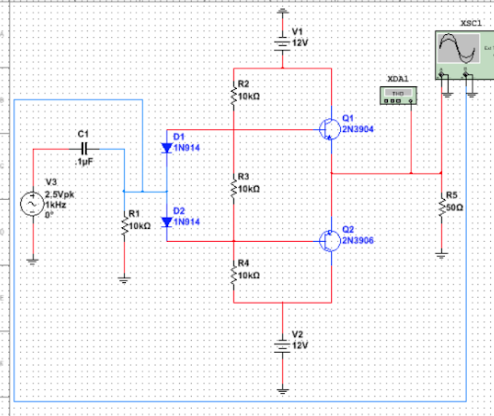Please complete analysis of the circuit above (diodes, current-mirrors, etc.). The circuit is a buffer-amplifier. Please include any appropriate calculations. V1 12V XDA R2 10kn 01 2N3904 C1 01 IN914 TuF R3 10kn V3 2.5Vpk R5 MkHz D2 RI 10kQ 1N014 02 2N3906 R4 V2 12V V1 12V XDA R2 10kn 01 2N3904 C1 01 IN914 TuF R3 10kn V3 2.5Vpk R5 MkHz D2 RI 10kQ 1N014 02 2N3906 R4 V2 12V